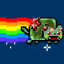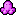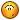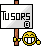Jagware## Recommended Posts

ggn    0

Hi,

```          r1 = grad1 And \$ff : r4 = grad4 And \$ff
g1 = Shr%(grad1, 8) And \$ff : g4 = Shr%(grad4, 8) And \$ff
b1 = Shr%(grad1, 16) And \$ff : b4 = Shr%(grad4, 16) And \$ff

col = (Shl&(r1, 8) And \$f800) + (Shl&(b1, 3) And \$7c0) + (Shr&(g1, 2))         'write beginning gradient (1)
stepr = (r4 - r1) / 15 : stepg = (g4 - g1) / 15 : stepb = (b4 - b1) / 15        'calculate steps

For l = 1 To 14
col = (Shl&(Round(r1 + stepr * l  / 8) And 255, 8 + 3) And \$f800) + (Shl&(Round(b1 + stepb * l / 8) And 255, 3 + 3) And \$7c0) + (Round(g1 + stepg * l / 4)  And \$3f)

Next l

col = (Shl&(r4, 8) And \$f800) + (Shl&(b4, 3) And \$7c0) + (Shr&(g4, 2))         'write final gradient (15)```

(so the code calculates the steps for r,g,b from the 8bit coefficients of grad1,grad4 and then creates the gradients by adding the step values to grad1's values, and then shifts them into place and masks them).

This nearly works, but there are some errors that are really visible on pure grey gradients; the green component seems to not keep in sync with the red and green, so some ugly stuff are being shown instead of shades of gray. From some reference palettes I have, I seem to be either spot-on or +/-1 when creating my palettes.

Anyone had any experiences with this? The only thing I wanted to try was to calculate the gradients using 16bit mode (5R,6G,5B) but I don't think that would change things much. Any input is appreciated!

##### Share on other sites

What I'd do is work on 8 bit values for R, G and B (24BPP) until you absolutely need to convert them to 16BPP mode then weight them accordingly (in a final pass). Are stepr, stepg, stepb floating point variables in your code?

##### Share on other sites

maybe it's because Green is 0-63 where as Red/Blue are 0-31.

For my palettes I personally keep 24bits colors and convert them on Jaguar in 16bits using a little 68k routine

##### Share on other sites
maybe it's because Green is 0-63 where as Red/Blue are 0-31.

I suggested this but maybe he'll listen if it's coming from someone like you rather than me##### Share on other sites

Well, I used to write code-that-does-everything-in-one-line like " col = (Shl&(Round(r1 + stepr * l / 8) And 255, 8 + 3) And \$f800) + (Shl&(Round(b1 + stepb * l / 8) And 255, 3 + 3) And \$7c0) + (Round(g1 + stepg * l / 4) And \$3f)"

but I find out that sometimes it led to strange behavior (missing parenthesis or compiler wrong interpretation)

Now I write a step each line, and then I can track the value after each computation and verify that I did everything right

just a suggestion %)

##### Share on other sites
ggn    0

Oops, people replied. I'm sorry but I've been busy with other parts of the tool so I didn't notice...

Now then...

What I'd do is work on 8 bit values for R, G and B (24BPP) until you absolutely need to convert them to 16BPP mode then weight them accordingly (in a final pass). Are stepr, stepg, stepb floating point variables in your code?

Yup that's what I do.

maybe it's because Green is 0-63 where as Red/Blue are 0-31.

For my palettes I personally keep 24bits colors and convert them on Jaguar in 16bits using a little 68k routine

Not an option here I'm afraid, a pre-computed palette is required.

maybe it's because Green is 0-63 where as Red/Blue are 0-31.

I suggested this but maybe he'll listen if it's coming from someone like you rather than meWell, I used to write code-that-does-everything-in-one-line like " col = (Shl&(Round(r1 + stepr * l / 8) And 255, 8 + 3) And \$f800) + (Shl&(Round(b1 + stepb * l / 8) And 255, 3 + 3) And \$7c0) + (Round(g1 + stepg * l / 4) And \$3f)"

but I find out that sometimes it led to strange behavior (missing parenthesis or compiler wrong interpretation)

Now I write a step each line, and then I can track the value after each computation and verify that I did everything right

just a suggestion %)

Yeah I know, I'm against franken-code like this, at the beginning it was a really simple line, but I kept adding stuff to it to see if it worked (as a proof-of-concept). I'll probably revisit that chunk of code tomorrow and make it less horrible!

Thanks for the tips, all (also on IRC)! Finally, for reference, here's a sample output for the r,g,b values using my routine (left) and the reference palette (right). I'll have another look tomorrow.

```0            39            15            0             39            15
0            36            14            0             37            14
0            34            13            0             33            12
0            32            12            0             31            11
0            30            11            0             29            09
0            28            10            0             27            08
0            26            9             0             25            07
0            24            8             0             23            06
0            22            7             0             21            05
0            19            6             0             19            04
0            17            5             0             17            03
0            15            4             0             15            02
0            13            3             0             13            02
0            11            2             0             11            01
0            9             1             0             9             01```

##### Share on other sites

There's something I don't understand with your code...

The gradient list is 15 entries long, but your code seems to generate 16 (1 + 14 + 1) entries :

``` 'write beginning gradient
...
For l = 1 To 14
...
Next l
...

If there are indeed 15 entries, then it needs a few patches :

```stepr = (r4 - r1) / 14 : stepg = (g4 - g1) / 14 : stepb = (b4 - b1) / 14
...
For l = 1 To 13```

##### Share on other sites

Just did a quick test, and here's something that seems to match the original gradient:

```DEFDBL A-Z

R1 = 0: G1 = 39: B1 = 15
R2 = 0: G2 = 9: B2 = 1

FOR I = 0 TO 14
R = FIX(R1 + (((R2 - R1) * I) / 14))
G = FIX(G1 + (((G2 - G1) * I) / 14))
B = FIX(B1 + (((B2 - B1) * I) / 14))
NEXT I```

And here are the RGB values it generates:

```0             39            15
0             36            14
0             34            13
0             32            12
0             30            11
0             28            10
0             26            9
0             24            8
0             21            7
0             19            6
0             17            5
0             15            4
0             13            3
0             11            2
0             9             1```

I don't have GFA32, so I tested it with gool old QBASIC. Note that FIX(x) always rounds down, not to the nearest integer (I tried adding .5 to round to the nearest integer, but it no longer matches the original gradient, though it's probably more accurate that way).

EDIT: oops, looks like what I thought was the original gradient is actually your version. Back to square one then...

EDIT 2 : If you look at the differences between successive colors, there's something strange in the original palette:

```0    -2    -1
0    -4    -2
0    -2    -1
0    -2    -2
0    -2    -1
0    -2    -1
0    -2    -1
0    -2    -1
0    -2    -1
0    -2    -1
0    -2    -1
0    -2    0
0    -2    -1
0    -2    0```

* the green channel uses -4 and -2, but not -3

* the blue channel uses more than two values (-2, -1, 0) and it doesn't look like there's a recurring pattern

IIRC that can't happen if the interpolation is linear, even with rounding. So either they were doing some really strange rounding, or they didn't use a linear interpolation (maybe it's gamma-corrected or picked manually?)

Is it important to match the original palette exactly?

##### Share on other sites
ggn    0

Sorry for delaying my reply so much - busy busy busy lately!

Since zerosquare's experiments confirm that the palettes are not exactly linear, I'll change strategies. Thanks to everyone here and on IRC for the suggestions and brainstorming.

##### Share on other sites×   Pasted as rich text.   Paste as plain text instead

Only 75 emoticons maximum are allowed.